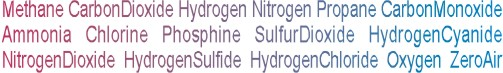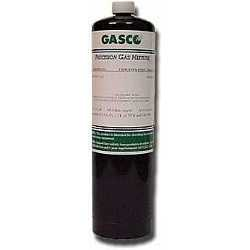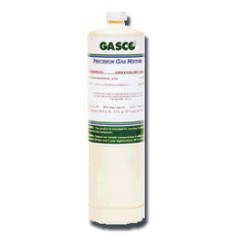Calibration gas blends, mixes and accessories
NON-REACTIVE SINGLE GAS > CARBON DIOXIDE :    48 Items found.(1047)

## NORLAB Equivalent  P101325N

Norlab Equivalent Calibration Gas 2.5% vol. Carbon Dioxide Balance nitrogen 17L Liter cylinder (Made by Gasco)

Configure

\$32.00(1035)

## NORLAB Equivalent  P1013100N

Norlab Equivalent Calibration Gas 100 ppm Carbon Dioxide Balance nitrogen 17L Liter cylinder (Made by Gasco)

Configure

\$32.00(1051)

## NORLAB Equivalent  P101315N

Norlab Equivalent Calibration Gas 15% vol. Carbon Dioxide Balance nitrogen 17L Liter cylinder (Made by Gasco)

Configure

\$32.00(1050)

## NORLAB Equivalent  P101310N

Norlab Equivalent Calibration Gas 10% vol. Carbon Dioxide Balance nitrogen 17L Liter cylinder (Made by Gasco)

Configure

\$32.00(1048)

## NORLAB Equivalent  P10135

Norlab Equivalent Calibration Gas 5% vol. Carbon Dioxide Balance air 17L Liter cylinder (Made by Gasco)

Configure

\$32.00(1046)

## NORLAB Equivalent  P10132N

Norlab Equivalent Calibration Gas 2% vol. Carbon Dioxide Balance nitrogen 17L Liter cylinder (Made by Gasco)

Configure

\$32.00(1045)

## NORLAB Equivalent  P10132

Norlab Equivalent Calibration Gas 2% vol. Carbon Dioxide Balance nitrogen 17L Liter cylinder (Made by Gasco)

Configure

\$32.00(1044)

## NORLAB Equivalent  P1013015N

Norlab Equivalent Calibration Gas 1.5% vol. Carbon Dioxide Balance air 17L Liter cylinder (Made by Gasco)

Configure

\$32.00(1037)

## NORLAB Equivalent  P1013500N

Norlab Equivalent Calibration Gas 500 ppm Carbon Dioxide Balance nitrogen 17L Liter cylinder (Made by Gasco)

Configure

\$32.00(1042)

## NORLAB Equivalent  P10132000N

Norlab Equivalent Calibration Gas 2000 ppm Carbon Dioxide Balance nitrogen 17L Liter cylinder (Made by Gasco)

Configure

\$32.00(1040)

## NORLAB Equivalent  P10131000N

Norlab Equivalent Calibration Gas 1000 ppm Carbon Dioxide Balance nitrogen 17L Liter cylinder (Made by Gasco)

Configure

\$32.00(1036)

## NORLAB Equivalent  P1013200N

Norlab Equivalent Calibration Gas 200 ppm Carbon Dioxide Balance nitrogen 17L Liter cylinder (Made by Gasco)

Configure

\$32.00(1039)

## NORLAB Equivalent  P10137800N

Norlab Equivalent Calibration Gas 800 ppm Carbon Dioxide Balance nitrogen 17L Liter cylinder (Made by Gasco)

Configure

\$32.00(1038)

## NORLAB Equivalent  P1013700N

Norlab Equivalent Calibration Gas 700 ppm Carbon Dioxide Balance nitrogen 17L Liter cylinder (Made by Gasco)

Configure

\$32.00(1043)

## NORLAB Equivalent  P101305N

Norlab Equivalent Calibration Gas 0.5% vol Carbon Dioxide Balance nitrogen 17L Liter cylinder (Made by Gasco)

Configure

\$32.00(1049)

## NORLAB Equivalent  P10135N

Norlab Equivalent Calibration Gas 5% vol. Carbon Dioxide Balance nitrogen 17L Liter cylinder (Made by Gasco)

Configure

\$32.00(1057)

## NORLAB Equivalent  HP10132000N

Norlab Equivalent Calibration Gas 2000 ppm Carbon Dioxide Balance nitrogen 34LS Liter cylinder (Made by Gasco)

Configure

\$65.00(1056)

## NORLAB Equivalent  HP10131000N

Norlab Equivalent Calibration Gas 1000 ppm Carbon Dioxide Balance nitrogen 34LS Liter cylinder (Made by Gasco)

Configure

\$65.00(1055)

## NORLAB Equivalent  HP1013700N

Norlab Equivalent Calibration Gas 700 ppm Carbon Dioxide Balance nitrogen 34LS Liter cylinder (Made by Gasco)

Configure

\$65.00(1054)

## NORLAB Equivalent  HP1013500N

Norlab Equivalent Calibration Gas 500 ppm Carbon Dioxide Balance nitrogen 34LS Liter cylinder (Made by Gasco)

Configure

\$65.00
Page: *1 | 2 | 3 | All